# Prime numbers Composite numbers Neither prime nor composite

• Slides: 19Prime numbers Composite numbers Neither prime nor composite How to know?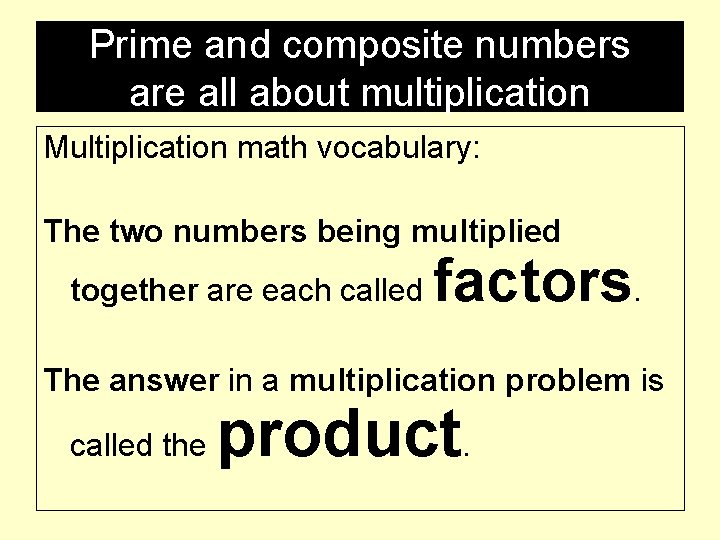Prime and composite numbers are all about multiplication Multiplication math vocabulary: The two numbers being multiplied together are each called factors. The answer in a multiplication problem is called the product.Multiplication factor x factor = product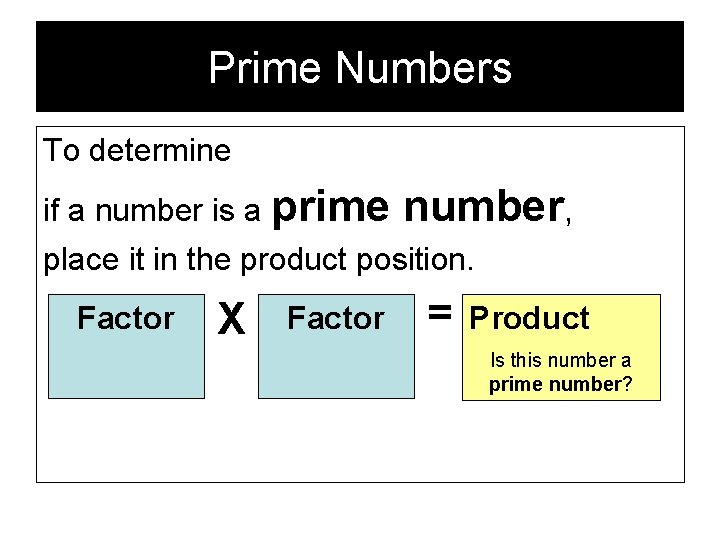Prime Numbers To determine if a number is a prime number, place it in the product position. Factor X Factor = Product Is this number a prime number?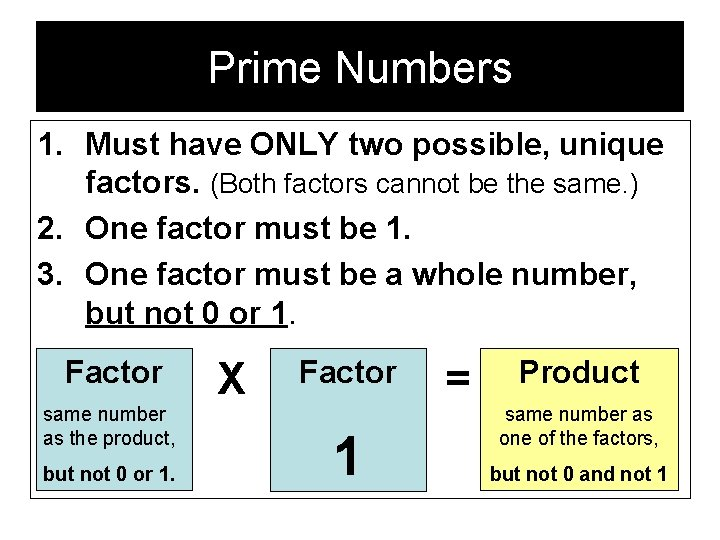Prime Numbers 1. Must have ONLY two possible, unique factors. (Both factors cannot be the same. ) 2. One factor must be 1. 3. One factor must be a whole number, but not 0 or 1. Factor same number as the product, but not 0 or 1. X Factor 1 = Product same number as one of the factors, but not 0 and not 1Whole numbers In case you forgot: Whole numbers are the number 0, and the natural numbers which start at positive one— {0, 1, 2, 3. . . }Prime Numbers 3 same number as the product, X 1 but not 0 or 1. = 3 same number as one of the factors, but not 0 and not 1 3 is a prime number. 1. There are ONLY two possible, unique factors. (No other two numbers multiplied together have a product of 3 and both factors are different numbers. ) 2. 3. One factor is 1. One factor is a whole number, but not 0 or 1.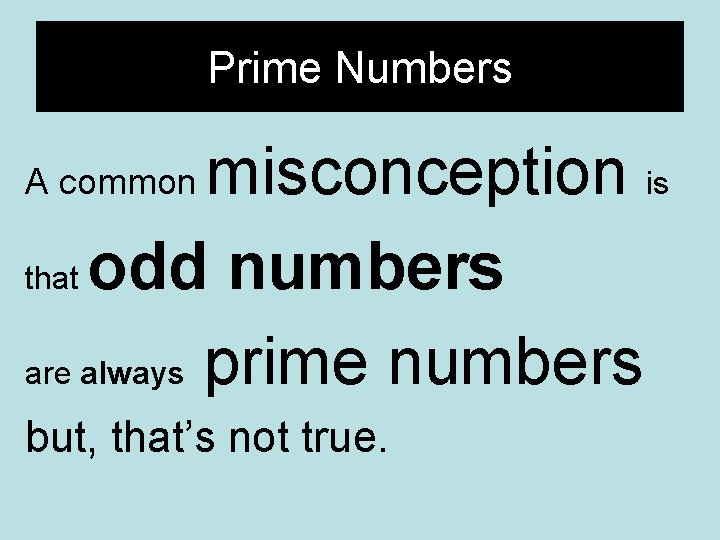Prime Numbers misconception is that odd numbers are always prime numbers A common but, that’s not true.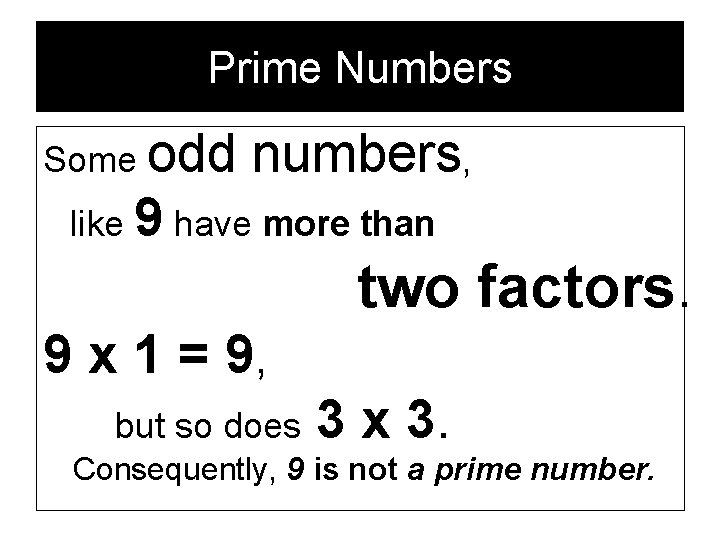Prime Numbers Some odd numbers, like 9 have more than two factors. 9 x 1 = 9, but so does 3 x 3. Consequently, 9 is not a prime number.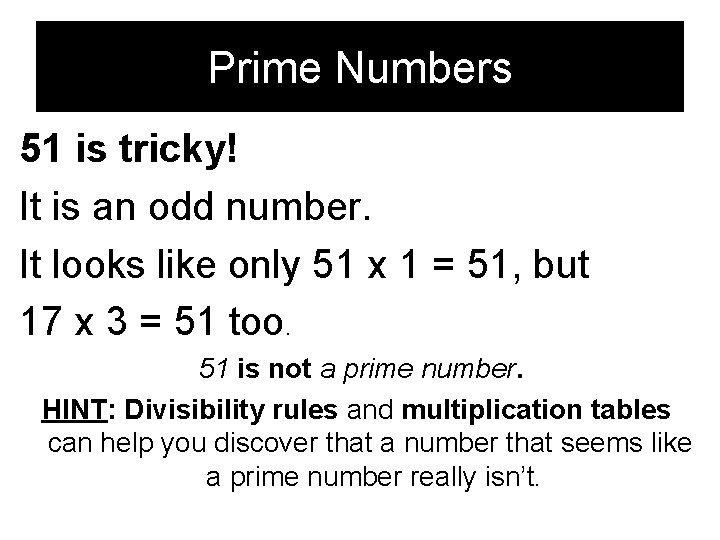Prime Numbers 51 is tricky! It is an odd number. It looks like only 51 x 1 = 51, but 17 x 3 = 51 too. 51 is not a prime number. HINT: Divisibility rules and multiplication tables can help you discover that a number that seems like a prime number really isn’t.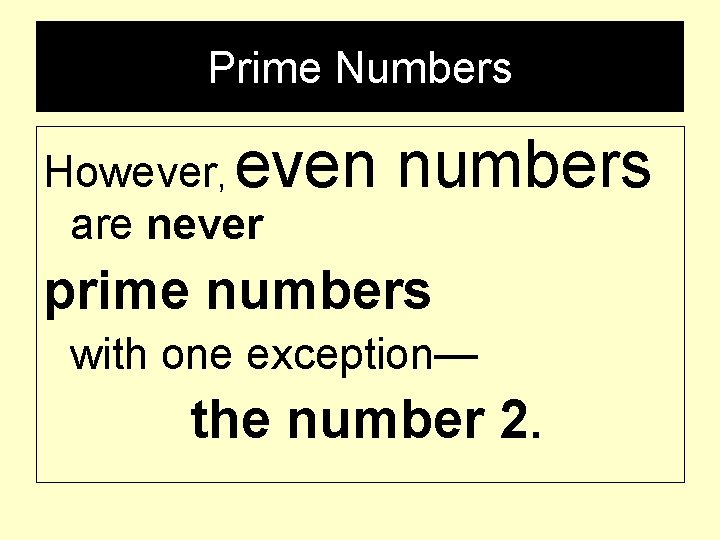Prime Numbers However, even are never numbers prime numbers with one exception— the number 2.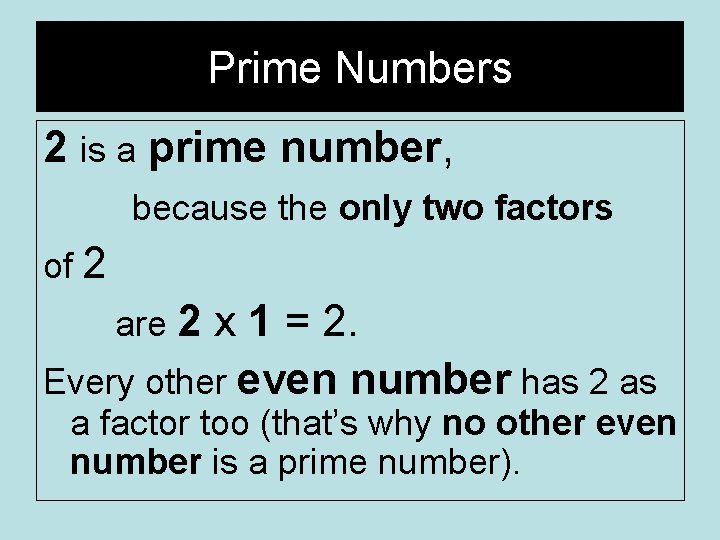Prime Numbers 2 is a prime number, because the only two factors of 2 are 2 x 1 = 2. Every other even number has 2 as a factor too (that’s why no other even number is a prime number).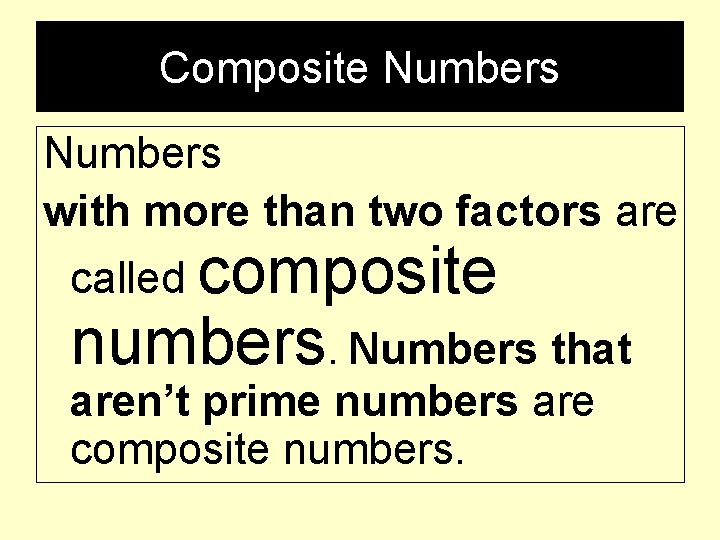Composite Numbers with more than two factors are called composite numbers. Numbers that aren’t prime numbers are composite numbers.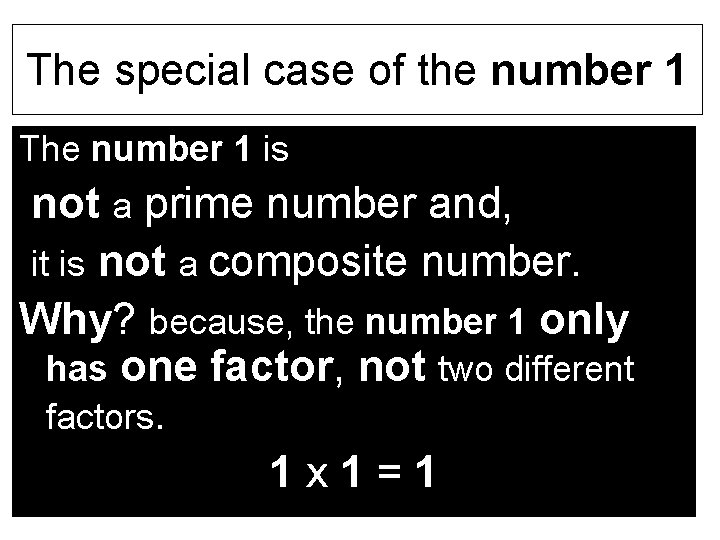The special case of the number 1 The number 1 is not a prime number and, it is not a composite number. Why? because, the number 1 only has one factor, not two different factors. 1 x 1=1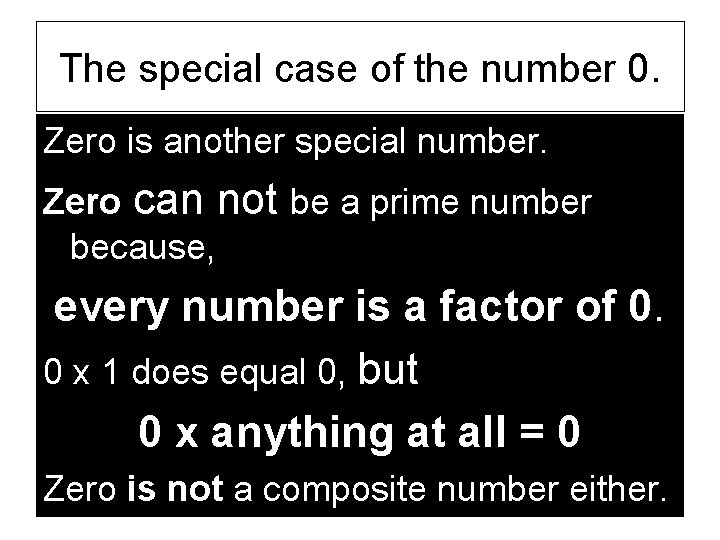The special case of the number 0. Zero is another special number. Zero can not be a prime number because, every number is a factor of 0. 0 x 1 does equal 0, but 0 x anything at all = 0 Zero is not a composite number either.Only 0 and 1 are neither prime nor composite numbers. All other whole numbers are either prime or composite numbers.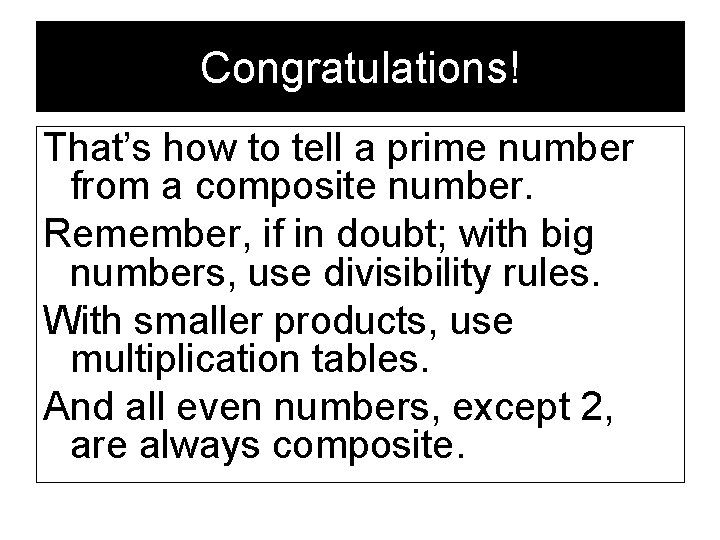Congratulations! That’s how to tell a prime number from a composite number. Remember, if in doubt; with big numbers, use divisibility rules. With smaller products, use multiplication tables. And all even numbers, except 2, are always composite.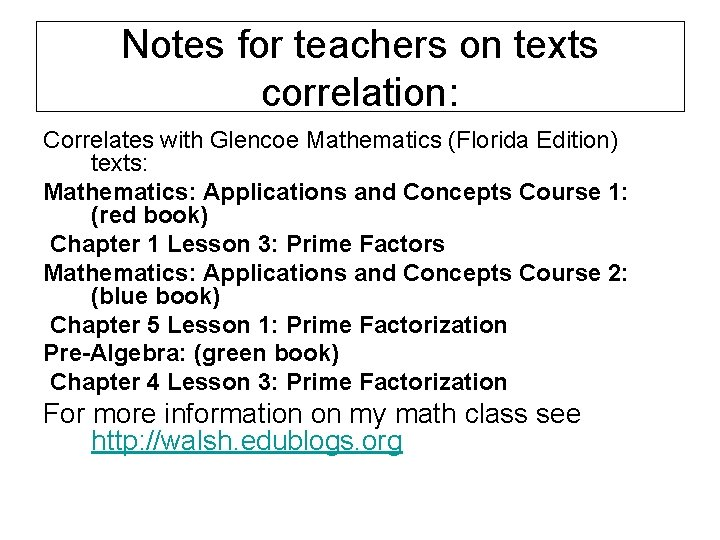Notes for teachers on texts correlation: Correlates with Glencoe Mathematics (Florida Edition) texts: Mathematics: Applications and Concepts Course 1: (red book) Chapter 1 Lesson 3: Prime Factors Mathematics: Applications and Concepts Course 2: (blue book) Chapter 5 Lesson 1: Prime Factorization Pre-Algebra: (green book) Chapter 4 Lesson 3: Prime Factorization For more information on my math class see http: //walsh. edublogs. orgNotes for teachers on design This slide presentation was created using Microsoft Office Power. Point 2003 part of Microsoft Office Standard Version for Students and Teachers. Finally, thank you. I hope this is of help to your students. Taleese